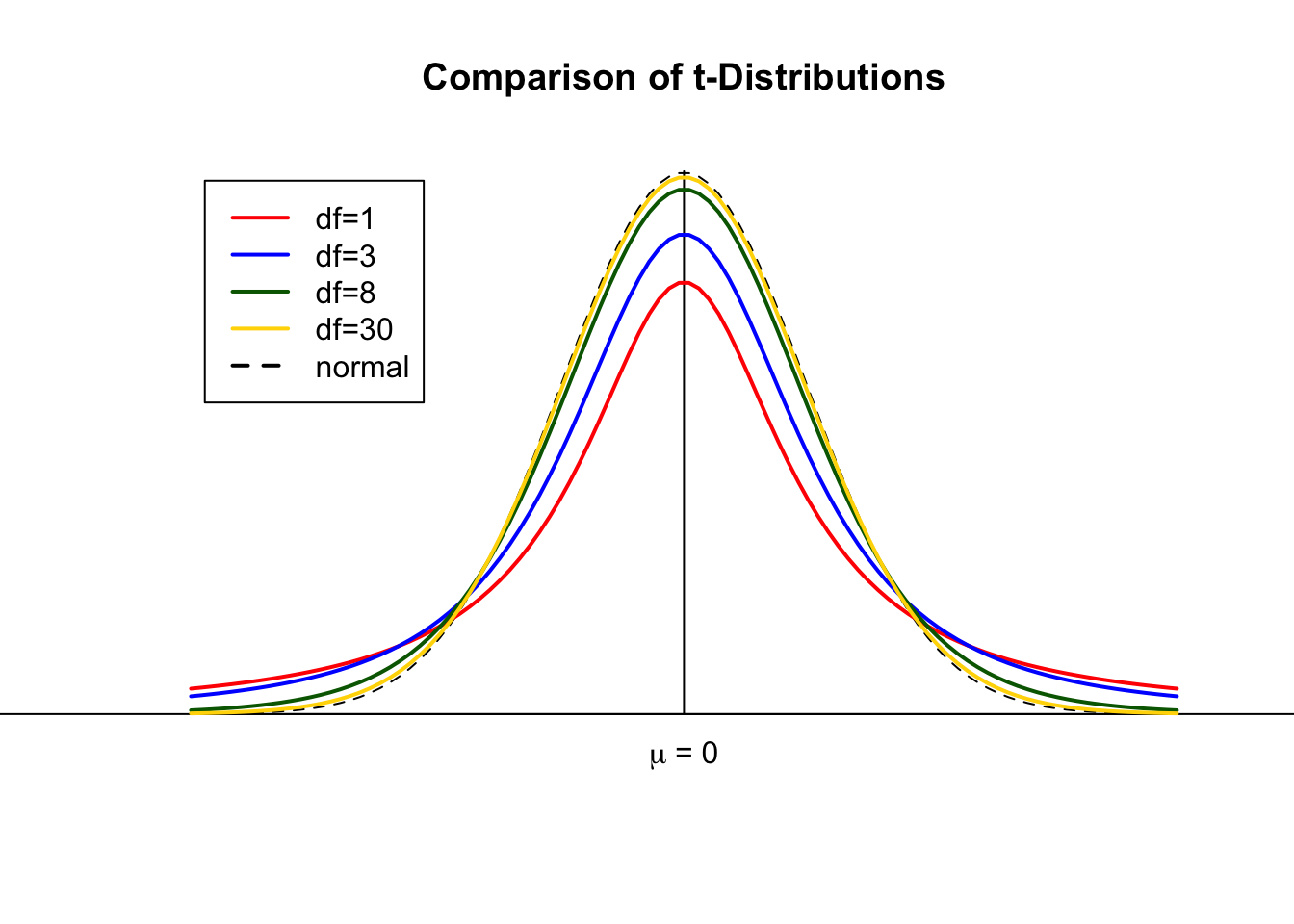Student’s t-distribution is named in honor of William Sealy Gosset (1876-1937), who first determined it in 1908. Gosset was one of the best Oxford graduates in chemistry and mathematics in his generation. In 1899, he took up a job as a brewer at Arthur Guinness Son & Co, Ltd in Dublin, Ireland. Working for the Guinness brewery, he was interested in quality control based on small samples in various stages of the production process. Since Guinness prohibited its employees from publishing any papers to prevent disclosure of confidential information, Gosset had published his work under the pseudonym “Student”, and his identity was not known for some time after the publication of his most famous achievements, so the distribution was named Student’s or t-distribution, leaving his name less well known than his important results in statistics (Lovric, 2010).

The t-distribution curve is, like the normal distribution curve, symmetric (bell shaped) about the mean. However, the t-distribution curve is flatter than the standard normal distribution curve. Consequently, the t-distribution curve has a lower height and a wider spread than the standard normal distribution.

The t-distribution has only one parameter, called the degrees of freedom $$(df)$$. The shape of a particular t-distribution curve depends on the number of degrees of freedom $$(df)$$. The number of degrees of freedom for a t-distribution is equal to the sample size minus one, that is,

$df = n - 1$

As the sample size, $$n$$, and thus $$df$$ increases, the t-distribution approaches the standard normal distribution. The units of a t-distribution are denoted by t. The mean of the t-distribution is equal to $$0$$, and its standard deviation is $$\sqrt{df/(df-2)}$$ (Mann, 2012).##### Basic Properties of t-curves (Weiss, 2010)
• The total area under a t-curve equals 1.
• A t-curve extends indefinitely in both directions, approaching, but never touching, the horizontal axis as it does so.
• A t-curve is symmetric about 0.
• As the number of degrees of freedom becomes larger, t-curves look increasingly like the standard normal curve.